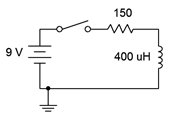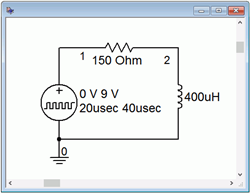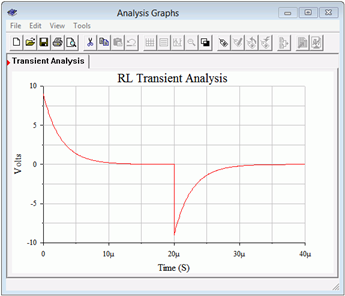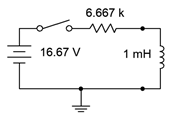# 6.2.5: Transient Response of RL Circuits

$$\newcommand{\vecs}{\overset { \rightharpoonup} {\mathbf{#1}} }$$ $$\newcommand{\vecd}{\overset{-\!-\!\rightharpoonup}{\vphantom{a}\smash {#1}}}$$$$\newcommand{\id}{\mathrm{id}}$$ $$\newcommand{\Span}{\mathrm{span}}$$ $$\newcommand{\kernel}{\mathrm{null}\,}$$ $$\newcommand{\range}{\mathrm{range}\,}$$ $$\newcommand{\RealPart}{\mathrm{Re}}$$ $$\newcommand{\ImaginaryPart}{\mathrm{Im}}$$ $$\newcommand{\Argument}{\mathrm{Arg}}$$ $$\newcommand{\norm}{\| #1 \|}$$ $$\newcommand{\inner}{\langle #1, #2 \rangle}$$ $$\newcommand{\Span}{\mathrm{span}}$$ $$\newcommand{\id}{\mathrm{id}}$$ $$\newcommand{\Span}{\mathrm{span}}$$ $$\newcommand{\kernel}{\mathrm{null}\,}$$ $$\newcommand{\range}{\mathrm{range}\,}$$ $$\newcommand{\RealPart}{\mathrm{Re}}$$ $$\newcommand{\ImaginaryPart}{\mathrm{Im}}$$ $$\newcommand{\Argument}{\mathrm{Arg}}$$ $$\newcommand{\norm}{\| #1 \|}$$ $$\newcommand{\inner}{\langle #1, #2 \rangle}$$ $$\newcommand{\Span}{\mathrm{span}}$$

The transient response of RL circuits is nearly the mirror image of that for RC circuits. To appreciate this, consider the circuit of Figure 9.5.1 .Figure 9.5.1 : RL circuit for transient response analysis.

Again, the key to this analysis is to remember that inductor current cannot change instantaneously. When power is first applied, the circulating current must remain at zero. Therefore no voltage drop is produced across the resistor, and by KVL, the voltage across the inductor must equal the source, $$E$$. This establishes the initial rate of change of current via Equation 9.2.9 $$(di/dt = E/L)$$ and is represented by the dashed red line in the graph of Figure 9.5.2 . As the current starts to increase, the voltage drop across the resistor begins to increase. This reduces the voltage available for the inductor, thus slowing the rate of change of current. This is depicted by the solid red curve on the graph. Meanwhile, the solid blue curve represents the decreasing inductor voltage. Thus, in the RL circuit, the inductor's voltage curve echoes the RC circuit's current curve (or resistor voltage curve), and the RL current curve echoes the RC circuit's capacitor voltage curve.

The curves presented in Figure 9.5.2 are identical to those presented in Chapter 8 when we discussed capacitors. They are reproduced here for your convenience.Figure 9.5.2 : Normalized charge and discharge curves.

As noted before, the rate of current change versus time is equal to $$\mathcal{v}/L$$, and therefore in this case, $$E/L$$. If the initial rate of change were to continue unabated, the maximum (steady-state) current, $$E/R$$, would be reached in $$L/R$$ seconds1. Therefore the time constant for an RL circuit is:

$\tau = \frac{L}{R} \label{9.13}$

Once again, five constants will achieve steady-state.

Following the prior work on capacitors, the relevant equations for the RL circuit can be shown to be2:

$V_L (t) = E \epsilon^{− \frac{t}{\tau}} \label{9.14}$

$V_R (t) = E \left(1 − \epsilon^{− \frac{t}{\tau}} \right) \label{9.15}$

$I (t) = \frac{E}{R} \left(1 − \epsilon^{− \frac{t}{\tau}} \right) \label{9.16}$

Where

$$V_L(t)$$ is the inductor voltage at time $$t$$,

$$V_R(t)$$ is the resistor voltage at time $$t$$,

$$I(t)$$ is the current at time $$t$$,

$$E$$ is the source voltage,

$$R$$ is the series resistance,

$$t$$ is the time of interest,

$$\tau$$ is the time constant,

$$\varepsilon$$ (also written $$e$$) is the base of natural logarithms, approximately 2.718.

Time for an example.

##### Example 9.5.1

Given the circuit of Figure 9.5.3 , assume the switch is closed at time $$t = 0$$. Determine the charging time constant, the amount of time after the switch is closed before the circuit reaches steady-state, and the inductor voltage and current at $$t = 0$$, $$t = 2$$ microseconds and $$t = 1$$ millisecond. Assume the inductor is initially uncharged.Figure 9.5.3 : Circuit for Example 9.5.1 .

First, the time constant:

$\tau = \frac{L}{R} \nonumber$

$\tau = \frac{400 \mu H}{150 \Omega} \nonumber$

$\tau \approx 2.667 \mu s \nonumber$

Steady-state will be reached in five time constants, or approximately 13.33 microseconds. Thus we know that $$V_L(0) = 9$$ volts and $$V_L(1 ms) = 0$$ volts. Because the inductor is an open initially, $$I_L(0) = 0$$ amps. At $$I_L$$(1 ms), the circuit is in steady-state and the inductor acts like a short. Therefore, all of the 9 volt source drops across the 150 $$\Omega$$ resistor, for 60 mA.

To find $$V_L$$(2 \mu s) we simply solve Equation \ref{9.14}.

$V_L (t) = E \epsilon^{− \frac{t}{\tau}} \nonumber$

$V_L (2 \mu s) = 9V \epsilon^{− \frac{2 \mu s}{2.667 \mu s}} \nonumber$

$V_L (2 \mu s) \approx 4.251 V \nonumber$

This value can also be determined graphically from Figure 9.5.2 . The time of 2 microseconds represents 75% of a time constant. Find this value on the horizontal axis and then track straight up to the solid blue curve that represents the charging inductor voltage. The point of intersection is right around 47% of the maximum value on the vertical axis. The maximum value here is the source voltage of 9 volts. Therefore the inductor will have reached approximately 47% of 9 volts, or just over 4.2 volts.

The current can be found in a similar manner using Equation \ref{9.16}.

$I_L (t) = \frac{E}{R} \left(1 − \epsilon^{− \frac{t}{\tau}} \right) \nonumber$

$I_L (2 \mu s) = \frac{9V}{150 \Omega} \left( 1 − \epsilon^{− \frac{2 \mu s}{2.667 \mu s}} \right) \nonumber$

$I_L (2 \mu s) = 60mA \left( 1 − \epsilon^{−0.75} \right) \nonumber$

$I_L (2 \mu s) = 31.66mA \nonumber$

## Computer Simulation

To verify our analysis, the circuit of Figure 9.5.3 is entered into a simulator, as shown in Figure 9.5.4 . In order to reflect the notion of a time-varying circuit with a switch, the 9 volt DC voltage source has been replaced with a rectangular pulse voltage source. This source starts at 0 volts and then immediately steps up to 9 volts. It stays at this level for 20 microseconds before dropping back to 0 volts.Figure 9.5.4 : The circuit of Figure 9.5.3 in a simulator.

The results of a transient analysis are shown in Figure 9.5.5 . The waveform shown tracks the inductor's voltage at node 2 with respect to ground.Figure 9.5.5 : Simulation results for the circuit of Figure 9.5.3 .

We can see that the voltage starts at 9 volts as expected. It then falls back to zero and is at steady-state in less than 15 microseconds, just as predicted. At 20 microseconds, the pulse source returns to zero volts. At this instant, the current through the inductor must still be the steady-state current of 60 milliamps. This current will still be flowing in a clockwise direction, thus it will produce a 9 volt drop across the 150 $$\Omega$$ resistor with a + to − polarity from left to right. This effectively places node 2 negative with respect to ground. The result is that the polarity of the inductor's voltage flips, with the inductor now acting as a short-lived source. We see this on the transient analysis as a negative 9 volt spike. The discharge time constant is identical to the charge constant, and thus we see the inductor's voltage fall back to zero in the same amount of time.

##### Example 9.5.2

Given the circuit of Figure 9.5.6 , find $$V_L$$ at $$t = 1$$ microsecond after the circuit is energized. Assume the inductor is initially uncharged.Figure 9.5.6 : Circuit for Example 9.5.2 .

First, the time constant:

$\tau = \frac{L}{R} \nonumber$

$\tau = \frac{6 mH}{15 k\Omega} \nonumber$

$\tau = 400 ns \nonumber$

Steady-state will be reached in five time constants, or 2 microseconds, at which point the inductor voltage will be zero as it will be behaving as a short. In contrast, as the inductor is initially an open $$(I_L(0) = 0)$$, all of the current from the source will flow into the 15 k$$\Omega$$ resistor, producing 30 volts across this parallel network. Therefore, we can state that $$V_L(0) = 30$$ volts and $$V_L$$(2 \mu s) = 0 volts, defining the extremes. In order to find $$V_L$$(1 \mu s), we can use Equation \ref{9.14}.

$V_L (t) = E \epsilon^{− \frac{t}{\tau}} \nonumber$

$V_L (1 \mu s) = 30 V \epsilon^{− \frac{1 \mu s}{0.4 \mu s}} \nonumber$

$V_L (1 \mu s) \approx 2.463 V \nonumber$

There are a few different ways to crosscheck this value. For starters, we can determine the inductor current using a slight modification of Equation \ref{9.16} (the current source value is used in place of $$E/R$$ as the equation effectively requires the maximum or steady-state current).

$I_L (t) = I \left( 1 − \epsilon^{− \frac{t}{\tau}} \right) \nonumber$

$I_L (1 \mu s) = 2mA \left( 1 − \epsilon^{− \frac{1 \mu s}{0.4 \mu s}} \right) \nonumber$

$I_L (1 \mu s) = 1.836mA \nonumber$

The inductor voltage of 2.463 volts would also have to appear across the parallel 15 k$$\Omega$$ resistor. This produces 2.463 V / 15 k$$\Omega$$, or 0.164 mA of resistor current. By KCL, the remainder of the 2 mA source current must be flowing down through the inductor. This yields a net inductor current of 2 mA − 0.164 mA, or 1.836 mA, verifying our prior result.

Example 9.5.2 also reinforces the concept of the time constant being inversely proportional to the resistance, rather than directly proportional as in the RC case. In the circuit of Figure 9.5.6 , it should be obvious that the larger the resistance value, the larger the resulting initial-state voltage. From Equation 9.2.9 it can be seen that if the voltage across the inductor is increased, then the initial rate of change of current with respect to time will increase, and that implies a shorter time constant.

For more complex circuits, Thévenin's theorem may be used in order to determine the effective source voltage and charging resistance. As we saw with RC circuits, it's also possible that the discharge resistance may be considerably different from the charging resistance. In such a case, the charge and discharge curves can be highly asymmetric in both time and amplitude. Generally, the larger the discharge resistance is when compared to the charge resistance, the larger in voltage and the shorter in time the discharge spike will be (think in terms of the area under the curve staying constant).

##### Example 9.5.3

Assume the initial current through the inductor is zero in Figure 9.5.7 . Determine the time constant. Also, determine the inductor voltage and the voltage across the 6 k$$\Omega$$ resistor 200 nanoseconds after the switch is closed.Figure 9.5.7 : Circuit for Example 9.5.3 .

This circuit is based on the circuit presented in Figure 9.3.2 as used in Example 9.3.1. In that analysis it was discovered that the steady-state voltage for the 6 k$$\Omega$$ and 2 k$$\Omega$$ resistors was 15 volts, the pair being in parallel. Further, the initial voltage across the 2 k$$\Omega$$ resistor and the inductor was 16.67 volts and for the 6 k$$\Omega$$ resistor, 0 volts.

For the Thévenin circuit, the open circuit voltage at the inductor would be the potential across the 2 k$$\Omega$$ resistor, which is obtained from a voltage divider between it and the 1 k$$\Omega$$ resistor, or 16.67 volts. The equivalent resistance is obtained by shorting the voltage source which leaves the 1 k$$\Omega$$ and 2 k$$\Omega$$ resistors in parallel, which is then in series with the 6 k$$\Omega$$ resistor, yielding approximately 6.667 k$$\Omega$$. The equivalent is shown in Figure 9.5.8 .Figure 9.5.8 : Thévenin equivalent of the circuit of Figure 9.5.7 driving the inductor.

Immediately, we can see that the initial-state voltage across the inductor is confirmed in the equivalent circuit. We can now determine the time constant.

$\tau = \frac{L}{R} \nonumber$

$\tau = \frac{1mH}{6.667 k \Omega} \nonumber$

$\tau = 150 ns \nonumber$

Steady-state will be reached in 750 nanoseconds. To find the inductor voltage we can use Equation \ref{9.14}.

$V_L (t) = E \epsilon^{− \frac{t}{\tau}} \nonumber$

$V_L (200 ns) = 16.67V \epsilon^{− \frac{200 ns}{150 ns}} \nonumber$

$V_L (200 ns) \approx 4.39 V \nonumber$

Referring back to the original circuit, in order to determine the voltage across the 6 k$$\Omega$$ resistor we can find the current through it and use Ohm's law. The current would be the same as the inductor's current as the two are in series. Thus, Equation \ref{9.16} would do the trick.

$I_L (t) = \frac{E}{R} \left( 1 − \epsilon^{− \frac{t}{\tau}} \right) \nonumber$

$I_L (200 ns) = \frac{16.67V}{6.667 k \Omega} \left( 1 − \epsilon^{− \frac{200 ns}{150 ns}} \right) \nonumber$

$I_L (200 ns) = 2.5mA \left( 1 − \epsilon^{−1.333} \right) \nonumber$

$I_L (200 ns) \approx 1.841mA \nonumber$

And finally,

$V_{6k} (200 ns) = I R \nonumber$

$V_{6k} (200 ns) = 1.841 mA 6 k \Omega \nonumber$

$V_{6k} (200 ns) \approx 11.05 V \nonumber$

Further, the voltage across the 2 k$$\Omega$$ resistor must be the sum, or approximately 15.44 volts.

## Computer Simulation

The results of Example 9.5.3 are crosschecked in a simulator. Once again the circuit is built using a pulse generator, as shown in Figure 9.5.9 .Figure 9.5.9 : Circuit of Figure 9.5.7 in a simulator.

A transient analysis is run out to 1 microsecond which is modestly into steady-state. Node voltages 2 and 3 are plotted, as shown in Figure 9.5.10 . The initial voltage across the 2 k$$\Omega$$ resistor (node 2) is as predicted, approximately 16.7 volts, and falls to 15 volts at steady-state, approximately 750 nanoseconds later. The voltage across the 6 k$$\Omega$$ resistor (node 2) starts at zero volts and also winds up at 15 volts in steadystate, just as predicted. Further, note that the predicted voltages across the 2 k$$\Omega$$ and 6 k$$\Omega$$ resistors at 200 nanoseconds are verified.Figure 9.5.10 : Transient analysis simulation of the circuit of Figure 9.5.7 .

The voltage across the inductor is node 2 minus node 3. This differential is plotted separately in Figure 9.5.11 . The expected initial, steady-state and 200 nanosecond voltages are as predicted.Figure 9.5.11 : Simulation of the inductor voltage versus time for the circuit of Figure 9.5.7 .

One very important observation is that if an RL circuit is abruptly altered or opened, very large voltage spikes may occur. This is due to the fact that inductor current cannot change instantaneously. If the circuit is opened, the open represents a very large resistance. Ohm's law indicates that the inductor current times this very large resistance may produce a very large voltage across the new open. In fact, the potential may be sufficient to cause a spark or arc. Note that because the current cannot change instantaneously (both magnitude and direction), the inductor now behaves as a voltage source of very high magnitude and with reverse polarity. This phenomenon is used to create the ignition spark in internal combustion engines. In short, the ignition coil is charged, creating some current flow. The circuit is then interrupted leaving just the coil in series with the spark plug, the spark plug being little more than a precisely sized gap between two electrodes. This results in a large voltage being developed across the spark plug gap, typically in the neighborhood of 20,000 volts, which is sufficient to create a small arc (i.e., the spark) that then ignites the air-fuel mixture in the piston.

An example of producing a discharge voltage spike that is considerably larger than the source voltage can be illustrated with the circuit of Figure 9.5.12 . We shall assume that the inductor is initially uncharged when power is applied, and that the switch is in position 1. In this case, the circuit consists of just the 12 volt source, the 2.2 k$$\Omega$$ resistor, and the inductor. The circuit reaches steady-state in roughly 227 nanoseconds. At that point the inductor behaves as a short, leaving the full 12 volt source to drop across the 2.2 k$$\Omega$$ resistor. This produces a clockwise current of approximately 5.455 mA.Figure 9.5.12 : Circuit illustrating large discharge voltage spike.

If we now move the switch to position 2, this current must be maintained because the current through the inductor cannot change instantaneously. The new discharge resistance is now the series combination of the two resistors, or 49.2 k$$\Omega$$. Ohm's law and KVL dictate that the resulting inductor voltage must be 49.2 k$$\Omega$$ times 5.455 mA, or just beyond −268 volts. This potential is negative because the clockwise current is flowing up through the 47 k$$\Omega$$ resistor, producing a drop + to − from ground up. The increased resistance also shortens the time constant and now steady-state will be reached in only 10.1 nanoseconds. Thus, we see a much larger magnitude spike (over twenty times that of the source voltage) with a much shorter time duration (less than one-twentieth of the charge time).

Depending on the kind of switch that is used, things can be even more extreme than what has been just described. Switches come in two basic varieties: make-beforebreak and break-before-make. The former makes contact with the second position before it breaks contact with the first position, while the latter does the opposite. The behavior just described assumes that a make-before-break switch is being used. In contrast, if a break-before-make switch is used, we'd see a drastically different result.

Let us pick up back at steady-state with 5.455 mA flowing through the inductor. We now throw the switch to position 2. This new switch breaks contact with the wire leading back to voltage source prior to it making contact with the 47 k$$\Omega$$ resistor. For a short instant of time the switch is not making contact with anything and the resulting resistance in the loop is determined by the air gap between the switch contacts. Even if this was a mere 10 M$$\Omega$$, the resulting potential would be over 50,000 volts. This will almost certainly create a spark and we will have inadvertently recreated the spark plug scenario. Hopefully, there are no combustible gases nearby.

## References

1Which is to say, $$E/L$$ amps per second times $$L/R$$ seconds yields $$E/R$$ amps.